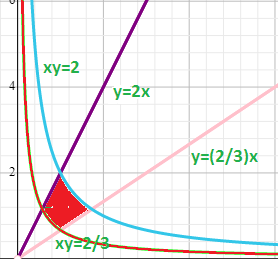# Use the given transformation to evaluate the integral: \iint_R 9xy \ dA , where R is the...

## Question:

Use the given transformation to evaluate the integral: {eq}\iint_R 9xy \ dA {/eq}, where {eq}R {/eq} is the region in the first quadrant bounded by the lines {eq}y= \frac {2}{3}x \ \text{and} \ y=2x {/eq} and the hyperbolas {eq}xy = \frac {2}{3} \ \text{and} \ xy=2; \ x = \frac{u}{v}, y=v {/eq}

## Double Integral:

In this problem, we have to evaluate the double integral by using the given transformation.

To evaluate the double integral, we use the formula of change of variable which is written as:

{eq}\iint_{D}f(x,y)dxdy=\iint_{S}f(g(u,v), h(u,v))\left | J \right |dudv {/eq}

where {eq}S {/eq} is the new bounded region after applying the given transformations and {eq}J {/eq} is the Jacobian that is defined as:

{eq}J =\dfrac{\partial (x,y)}{\partial (u,v)}=\begin{vmatrix} \dfrac{\partial x}{\partial u} & \dfrac{\partial x}{\partial v} \\ \dfrac{\partial y}{\partial u} & \dfrac{\partial y}{\partial v} \end{vmatrix}\\ {/eq}

We have to solve the integral {eq}\int \int_R 9xy dA, {/eq}

where R is the region in the first quadrant bounded by the lines {eq}y = \dfrac{2}{3}x {/eq} and {eq}y = 2x {/eq} and the hyperbolas {eq}xy = \dfrac{2}{3} {/eq} and {eq}xy=2 {/eq}

Given transformation {eq}x = \dfrac{u}{v}, y = v {/eq}The limits becomes:

{eq}\dfrac{2}{3}\leq u\leq 2\\ \sqrt{\dfrac{2u}{3}}\leq v\leq \sqrt{2u} {/eq}

To evaluate the integral, we use the following formula

{eq}\iint_{D}f(x,y)dxdy=\iint_{S}f(g(u,v), h(u,v))\left | J \right |dudv {/eq}

Now, we calculate the jacobian.

{eq}\begin{align} J &=\dfrac{\partial (x,y)}{\partial (u,v)}=\begin{vmatrix} \dfrac{\partial x}{\partial u} & \dfrac{\partial x}{\partial v} \\ \dfrac{\partial y}{\partial u} & \dfrac{\partial y}{\partial v} \end{vmatrix}\\ &=\begin{vmatrix} \dfrac{1}{v} & \dfrac{-u}{v^2}\\ 0 & 1 \end{vmatrix}\\ &=\dfrac{1}{v} \end{align} {/eq}

Therefore

{eq}\begin{align} \int \int_R 9xy dA,&=\int_{\dfrac{2}{3}}^{2}\int_{\sqrt{\frac{2u}{3}}}^{\sqrt{2u}}9\left ( \dfrac{u}{v} \right )(v)\left ( \dfrac{1}{v} \right )dvdu\\ &=\int_{\dfrac{2}{3}}^{2}\int_{\sqrt{\frac{2u}{3}}}^{\sqrt{u}}9\left ( \dfrac{u}{v} \right )dvdu\\ &=\int_{\dfrac{2}{3}}^{2}9u\left (\int_{\sqrt{\frac{2u}{3}}}^{\sqrt{2u}} \dfrac{1}{v}dv \right )du\\ &=\int_{\dfrac{2}{3}}^{2}9u\left ( \ln v \right )_{\sqrt{\frac{2u}{3}}}^{\sqrt{2u}}du\\ &=\int_{\dfrac{2}{3}}^{2}9u\left ( \ln \sqrt{2u}-\ln \sqrt{\dfrac{2u}{3}} \right )du\\ &=\int_{\dfrac{2}{3}}^{2}9u\left ( \dfrac{1}{2}\ln 2u-\dfrac{1}{2}\left ( \ln (2u)-\ln 3 \right ) \right )du\\ &=\int_{\dfrac{2}{3}}^{2}9u\left ( \dfrac{1}{2}\ln 2u-\dfrac{1}{2}\ln 2u+\dfrac{1}{2}\ln 3 \right )\\ &=\int_{\dfrac{2}{3}}^{2}9u\left ( \dfrac{1}{2}\ln \left ( 3 \right ) \right )du\\ &=\dfrac{9}{2}\ln \left ( 3 \right )\int_{\dfrac{2}{3}}^{2}udu\\ &=\dfrac{9}{2}\ln \left ( 3 \right )\left ( \dfrac{u^2}{2} \right )_{\dfrac{2}{3}}^{2}\\ &=\dfrac{9}{4}\ln \left ( 3 \right )\left ( u^2 \right )_{\dfrac{2}{3}}^{2}\\ &=\dfrac{9}{4}\ln \left ( 3 \right )\left ( 2^2-\left ( \dfrac{2}{3} \right )^2 \right )\\ &=\dfrac{9}{4}\ln \left ( 3 \right )\left ( 4-\dfrac{4}{9} \right )\\ &=\dfrac{9}{4}\ln \left ( 3 \right )\left ( \dfrac{32}{9} \right )\\ &=8\ln \left ( 3 \right ) \end{align} {/eq}